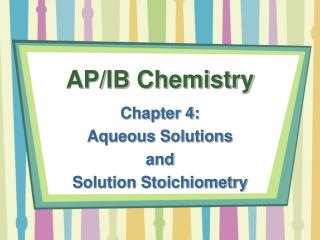DownloadDownload PresentationAP/IB Chemistry

# AP/IB Chemistry

Download Presentation## AP/IB Chemistry

- - - - - - - - - - - - - - - - - - - - - - - - - - - E N D - - - - - - - - - - - - - - - - - - - - - - - - - - -
##### Presentation Transcript

1. AP/IB Chemistry Chapter 4: Aqueous Solutions and Solution Stoichiometry

2. Definitions • Solution: homogenous mixture of • Solvent: • Present in greater quantities • Solute: • Aqueous Solution: solution in which • Solubility: amount of substance that dissolves in a

3. Ionic Compounds in Water • Solvation: the clustering of solvent molecules around a solute particle • Ions are solvated by water • Why? • Solvation of ions by water prevents anions and cations from • Why?

4. Solvation in Water

5. In-Class Problem Solvation of Ionic Compounds • What dissolved species are present in a solution of: • KCN • NaClO4 • K3PO4

6. Electrolytes vs. Nonelectrolytes • Electrolyte: substance that dissociates • Nonelectrolyte: substance that • 3 types of solutes in aqueous solutions:

7. Electrolytes as Conductors • Aqueous solutions can • Depends on number of • Transport of ions through solution causes

8. Molecular Compounds in Water • Aqueous solutions of molecular compounds do not • Ex: • If no ions in solution, • But… some molecular compounds dissociate into ions in aqueous solutions:

9. Strong and Weak Electrolytes • Strong Electrolytes: completely dissociate in solution • STRONG acids and bases (considered molecular compounds) • Weak Electrolytes: yield small concentration of ions when dissolved and mostly remain in molecular form • Ions exist in • Weak acids and bases

10. Concentrations of Solutions • Concentration: amount of solute dissolved in a • Change amounts of to change concentration • Measures of solution concentration • Molarity: moles of solute per • Units: • Molality: moles of solute per • Units: mol/kg

11. In-Class Problem Calculating Molarity • Calculate the molarity of a solution made by dissolving 5.00 g of glucose in 100 mL of water.

12. In-Class Problems Calculating Molar Concentration of Ions • What is the molar concentration of K+ ions in a 0.015 M solution of potassium chloride? • What is the molar concentration of K+ ions in a 0.015 M solution of potassium carbonate? • Why?

13. In-Class Problems • How many grams of sodium sulfate are there in 15 mL of 0.50 M sodium sulfate? • How many mL of 0.50 M sodium sulfate solution are needed to provide 0.038 mol of this salt? • What volume of 2.50 M lead (II) nitrate solution contains 0.050 mol of nitrate? • What volume of 2.50 M lead (II) nitrate solution contains 1.30 g of Pb2+?

14. Dilutions • A solution of lower concentration is obtained by • Key: Number of moles in both concentrated ( ) solution and ( ) solution

15. In-Class Problems Diluting Solutions • How many mL of a 5.0 M K2Cr2O7 solution must be diluted to prepare 250 mL of a 0.10 M solution? • If 10.0 mL of a 10.0 M stock solution of NaOH is diluted to 250 mL, what is the concentration of the resulting solution?

16. Solution Stoichiometry

17. In-Class Problems Solution Stoichiometry • How many grams of sodium hydroxide are needed to neutralize 20.0 mL of a 0.150 M sulfurous acid solution? • How many liters of a 0.50 M HCl solution are needed to react completely with 0.100 mol of lead(II) nitrate, forming a precipitate of lead(II) chloride?

18. Titrations

19. Titrations Suppose we know the concentration of a NaOH solution and we want to find the concentration of a HCl solution • We know: • What do we want? • What do we do? • Take a known volume of the

20. Titrations • What do we get? • Volume of • We know molarity of , so we can calculate moles of • Next step? • We also know • We can calculate moles of • Can we finish? • Knowing moles of and volume of we can calculate

21. In-Class Problems Acid-Base Titrations • What is the molarity of an sodium hydroxide solution if 48.0 mL is needed to neutralize 35.0 mL of 0.144 M carbonic acid? • 45.7 ml of 0.500 M sulfuric acid is required to neutralize a 20.0 mL sample of potassium hydroxide solution. What is the concentration of the potassium hydroxide solution?

22. In-Class Problem Redox Titrations • An iron ore is dissolved in acid and the iron is converted to Fe2+. The sample is then titrated with 47.20 mL of 0.02240 M MnO4- solution. The redox reaction that occurs during titration is as follows: MnO4-(aq) + 5Fe2+(aq) + 8H+(aq) Mn2+(aq) + 5Fe3+(aq) + 4H2O(l) • How many moles of MnO4- were added to the solution? • How many moles of Fe2+ were in the sample? • How many grams of iron were in the sample? • If the sample had a mass of 0.8890 g, what is the percentage of iron in the sample?

23. Sample Test Question 70.5 mg of potassium phosphate is added to 15.0 mL of 0.050 M silver nitrate solution, resulting in the formation of a precipitate. • Write the molecular equation for the reaction. • What is the limiting reagent in the reaction? • Calculate the theoretical yield, in grams, of the precipitate that forms.